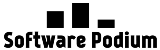# Website Conversion Rate Calculator

#### Conversion Rate:

Conversion rates serve as a critical element in online advertising and marketing. That’s why we’ve created this free website conversion rate calculator.

Measuring these rates is a fundamental step in determining the success of online campaigns. Whether it’s Email Marketing campaigns, Social Media, or PPC, this guide will elaborate on conversion rates, how to calculate them, and why they are indispensable for online business triumph.

## What is Conversion Rate?

The conversion rate is a fraction of your website’s visitors who take a desired action, such as filling out a form or purchasing. Calculating this rate is a pivotal approach to measuring the efficacy of your online campaigns and highlighting areas that need improvement.

## Why is the Calculation of Conversion Rates So Important?

Familiarizing yourself with conversion rates enables you to assess the efficiency of your online marketing efforts and determine areas where your campaigns are succeeding and areas that require improvement. Analysis of your conversion rates leads to data-driven decisions that enhance the profitability of your online business.

## How To Calculate Conversion Rate?

To calculate your conversion rate, divide the number of conversions by the number of visitors *100. Below, we have included a conversion rate calculator tool that simplifies calculating your conversion rate.

Conversion rate = (Conversions / Total visitors) x 100%

## Factors Influencing Conversion Rates

Several factors contribute to the variance of your conversion rate. The design of your website, the quality of your traffic, and the efficiency of your marketing campaigns are just a few examples. Understanding these factors and their impacts on your conversion rate will help you refine your website and marketing strategies to boost your conversion rates.

The conversion rate formula for Google Ads is the same as for any other advertising platform. You divide the number of conversions by the number of clicks and then multiply by 100 to get the conversion rate percentage. So the formula is (Conversions / Clicks) x 100 = Conversion Rate.

As mentioned above, the formula is the same for every platform (Facebook included). You divide the number of conversions by the number of clicks or impressions, depending on how you track conversions, and then multiply by 100 to get the conversion rate percentage. So the formula is (Conversions / Clicks or Impressions) x 100 = Conversion Rate.

## Website Conversion Rate Calculator FAQS

What is a good conversion rate?

A good conversion rate varies depending on the industry, product, and campaign but generally falls between 2-5%.

How to calculate conversion rate in e-commerce?

Divide the number of orders by the number of visitors and multiply by 100 to get the conversion rate percentage.

How to calculate conversion rate in Excel?

Enter the number of conversions and the total number of visitors in two cells, then use the formula “=conversions/visitors*100” to calculate the conversion rate.

Why is my conversion rate so low?

There could be several reasons why your conversion rate is low, including poor website design, ineffective targeting, or unoptimized ad copy. Analyze your campaign and make adjustments to improve conversion rates.Enable registration in settings - general